# Lab 8: Trimeshes

Github Classroom assignment

Please put your answers to written questions in this lab, if any, in a Markdown file named README.md in your lab repo.

## Introduction

Welcome to lab 8!

At this point in the semester, you must've had some experience with implicit shapes. These represent surfaces via a set of constraints (i.e. equations and inequalities), such that all points which satisfy these constraints lie on the surface of the shape. That's rather indirect!

In this lab, we will learn a different representation of surfaces—triangle meshes, or trimeshes for short—which more explicitly describes points on a surface. We will spend some time today understanding what they are, how they work, and how we can generate our own.

### Objectives

1. Learn about trimeshes and how they are used to approximate an object's surface;
2. Implement generators for cube and sphere trimeshes at varying levels of detail.

## Conceptual Background

### Objects As Trimeshes

Most objects can be represented as surfaces, which can, in turn, be approximated by a collection of connected triangles. We call such a representation of an object a trimesh:

Extra: can all objects be represented as trimeshes?

Fog, clouds, and smoke cannot easily be represented as surfaces, and are thus typically not represented as trimeshes.

In these cases, we instead rely on volumetric representations. If you're interested, you can read more about how such objects are rendered, through a process known as volumetric rendering.

### Level Of Detail (Tessellation Parameters)

As the number of triangles used in a trimesh (i.e. its tri-count) increases, the mesh looks more and more like the surface it is approximating. However, the cost of rendering it also increases.

To manage this tradeoff, we often vary a mesh's tri-count based on how much detail we want in the resulting 3D shape. This is loosely expressed as the trimesh's level of detail, which can be captured in the form of one or more tessellation parameters.Figure 2: A face represented by a triangle mesh, at increasing levels of detail.

### Single Triangles

Each triangle in a trimesh is defined by its three vertices, which may have attributes like positions, normals, or colors. First, let's consider only their positions.

#### Positions

We can store a triangle's vertices' positions in a vector, adopting a counter-clockwise winding order by convention. This simply means that we list the vertices in counter-clockwise order as viewed from the "front" of the triangle.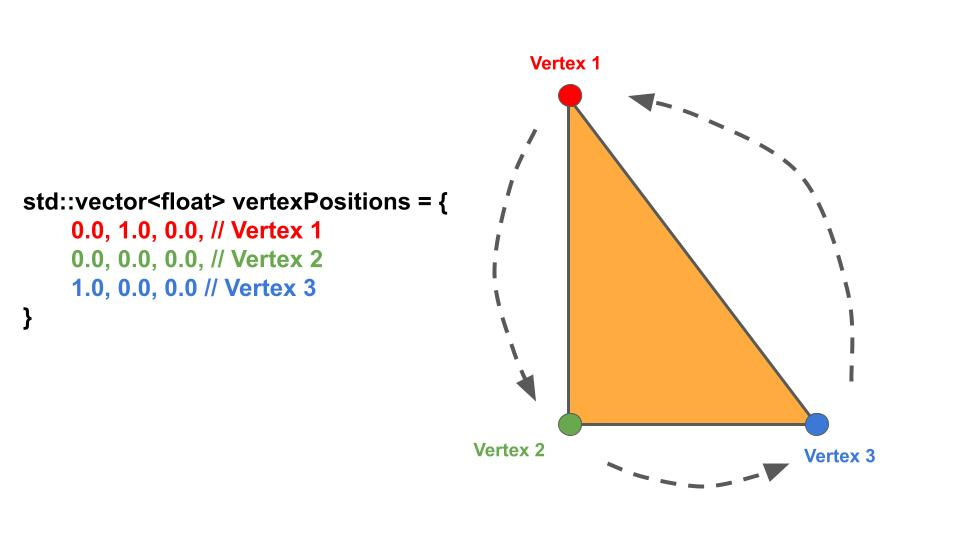Figure 3: A triangle's vertices' positions can be listed in a vector, in counter-clockwise order.

#### Normals

If we're alright with every point on our triangle having the same normal, we can use per-face normals. With three vertices, we can get two vectors; taking the cross product of these gives us the triangle's normal.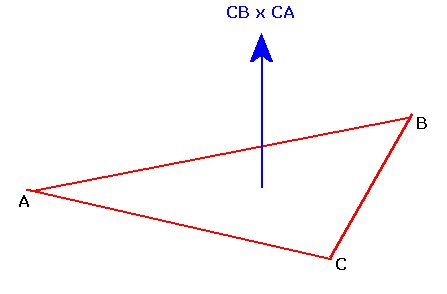Figure 4: A triangle's normal can be computed with just its vertices' positions.

However, we usually want points on our triangle to have different, spatially-varying normals, so that our lighting can (eventually) appear smoother. In such cases, we'd instead use per-vertex normals.

For our purposes in CS 1230, we will be using per-vertex normals.

Warning: observe how the tip of the cone in the image above has many normals defined for what appears to be the same vertex. This highlights a critical distinction: by "per-vertex", we don't mean "per-spatial-location", we mean "per-vertex-per-triangle". Many triangles have a vertex located at the tip of the cone, and each of those vertices can have a unique normal.

#### Positions And Normals

Putting it all together, we can represent each triangle vertex with 6 float values: for its position, and for its normal.

Seeing that each triangle has three vertices, and recalling that we're using a counter-clockwise winding order, we can derive something like m_vertexData below:

Extra: why does winding order matter?

In all subsequent assignments in CS 1230, you will need to use the correct winding order of vertex attributes (e.g. positions and normals) to be able to properly render anything.

This is because, as one of the real-time pipeline's many optimizations, backface culling is enabled by default. This simply means that the "back" faces of each triangle won't be rendered:

### Multiple Triangles

To represent a collection of triangles, we simply append the information for each new triangle to the back of our earlier list, i.e.. m_vertexData. Notice that:

• Positions and normals are interleaved, just as before; and
• Positions might be repeated, which is totally fine—multiple triangles can reasonably share the same position for some of their vertices.

### Summary

To summarize the above:

1. An object can be represented as a number of triangles, varying with its level of detail; and
2. A collection of triangles can be represented as a list of interleaved positions and normals.

## Getting Started

With that out of the way, we're finally at this lab's overarching "super-task":

You will implement two "trimesh generators", one for unit cubes and another for unit spheres. These should take in some tessellation parameters, then produce lists of vertex data.

### Stencil Code

Take a look at our stencil code. The only files you need to concern yourself with in this lab are Triangle.cpp, Cube.cpp, and Sphere.cpp, each located in the src/shapes/ directory.

There are two additional files in that directory: Cylinder and Cone. These can be ignored for now, but you may use them in Project 5.

Each shape class contains a member variable std::vector<GLfloat> m_vertexData. Note: a GLFloat is just a float by a different name.

In the body of each shape class' setVertexData() function, you will populate m_vertexData with the positions and normals of your shape's vertices, based on the tessellation parameters provided in m_param1 and m_param2. These tessellation parameters correspond to the parameter sliders in the application window.

### The Application Window

When you first run the program, you'll see a GUI pop-up that looks like this:

On the left sidebar, there are toggles to change the shape and sliders to change tessellation parameters 1 and 2. These control the level of detail of each shape—we'll provide more detail about these later in the handout.

On the right side of the GUI, you will eventually see the shapes generated from m_vertexData. You won't see anything right now because m_vertexData is empty.

### Drawing A Triangle

In Triangle.cpp, fill out the setVertexData() function. This function should update m_vertexData with positions and normals. Remember that the normals must be unit vectors.

For your triangle's vertices, please use these positions (listed in no particular order):

1. (-0.5, 0.5, 0.0)
2. (-0.5, -0.5, 0.0)
3. (0.5, -0.5, 0.0)

Which of these positions correspond to which points on the triangle? You don't have to write this one down, but you should be able to answer this question with ease.

Your triangle should look like this:Figure 10: Expected output of drawing a triangle to the screen.

Observe that if you click and drag to spin the triangle around, it'll disappear at certain angles. This is backface culling! Refer to the dropdown in this section on why winding order matters.

## Cubes

Now that you know how to create a triangle, it's time to render a cube :)

This cube's dimensions are identical to those of the implicit cube from your raytracer. It is centered at the origin, has a side length of 1, and is axis-aligned.

### Tiles

To create our cube, you'll first create a "tile": a plane comprising two triangles.

In Cube.cpp:

1. Uncomment the makeTile() function call in setVertexData().
2. Implement the makeTile() function. This function should update m_vertexData with a plane made of two triangles created from the input points.
• Use what you learned from making a triangle to do this!
• You will use this function for the next task.

You may find the following glm functions helpful:

• glm::vec3 glm::normalize(glm::vec3 v);
• glm::vec3 glm::cross(glm::vec3 v1, glm::vec3 v2);

Your tile should look like this: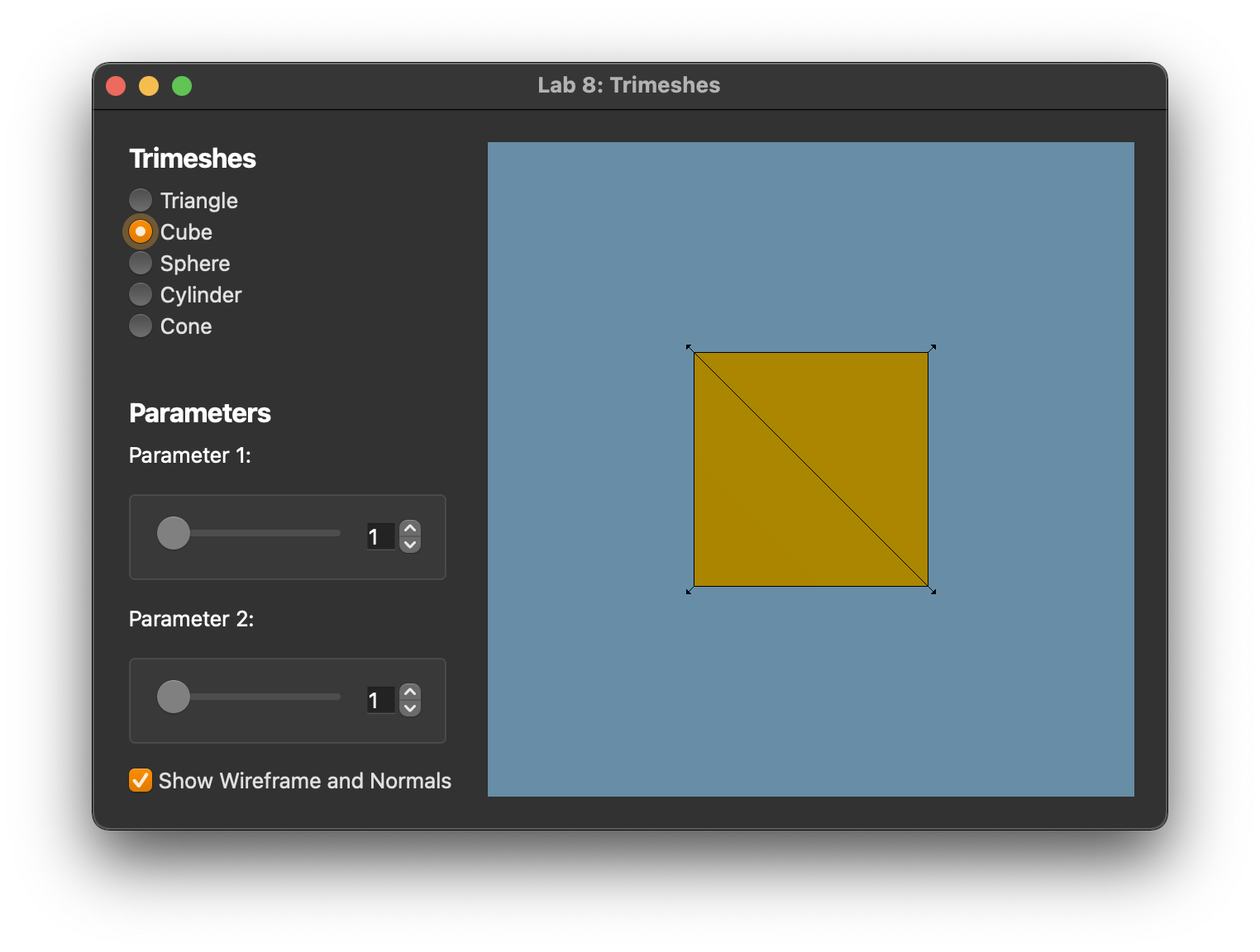Figure 11: Expected output of drawing a tile to the screen

### Cube Faces

Now that you can make individual tiles, you can tessellate a cube face!

Observe how parameter 1 controls the number of tiles in each row and column: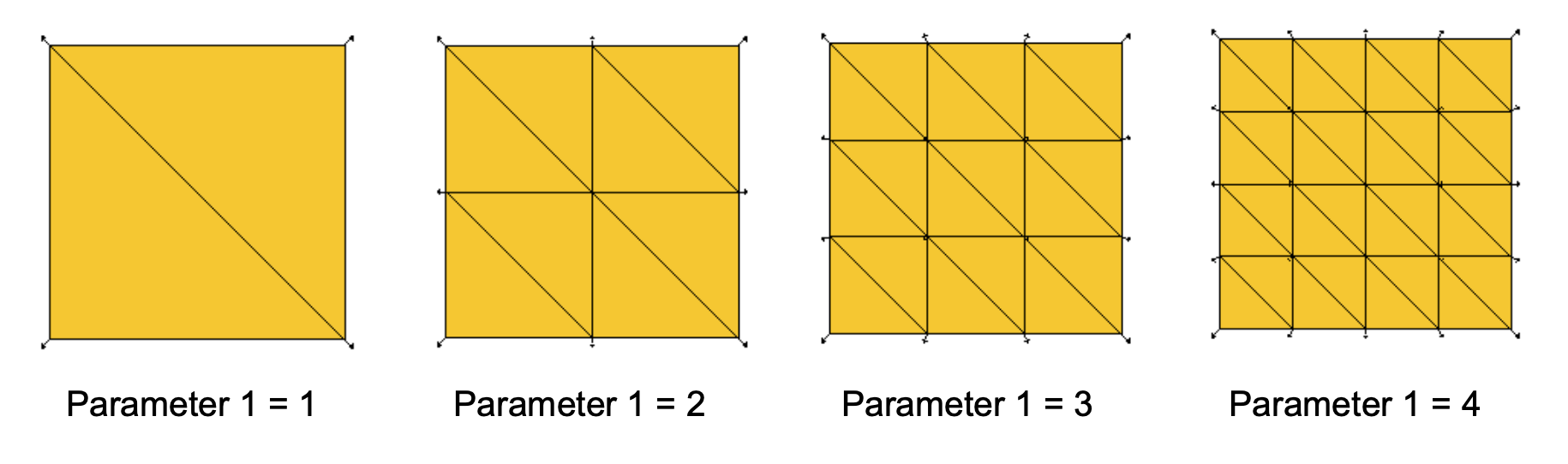Figure 12: Cube faces are increasingly tessellated at higher levels of detail.

In Cube.cpp

1. Comment out the makeTile() function call in setVertexData() and uncomment the makeFace() function call.
2. Complete the makeFace() function using the makeTile() function you wrote in the previous task. m_param1 contains the parameter 1 value.

Your face should look like this:Figure 13: Expected output of drawing a cube face to the screen, at different levels of detail.

### Full Cubes

Implement the remaining 5 sides of your Cube using the makeFace() function you implemented in the previous task.

It may be helpful to draw a diagram of the Cube to figure out the positions of all its corners!

Your cube should now look like this: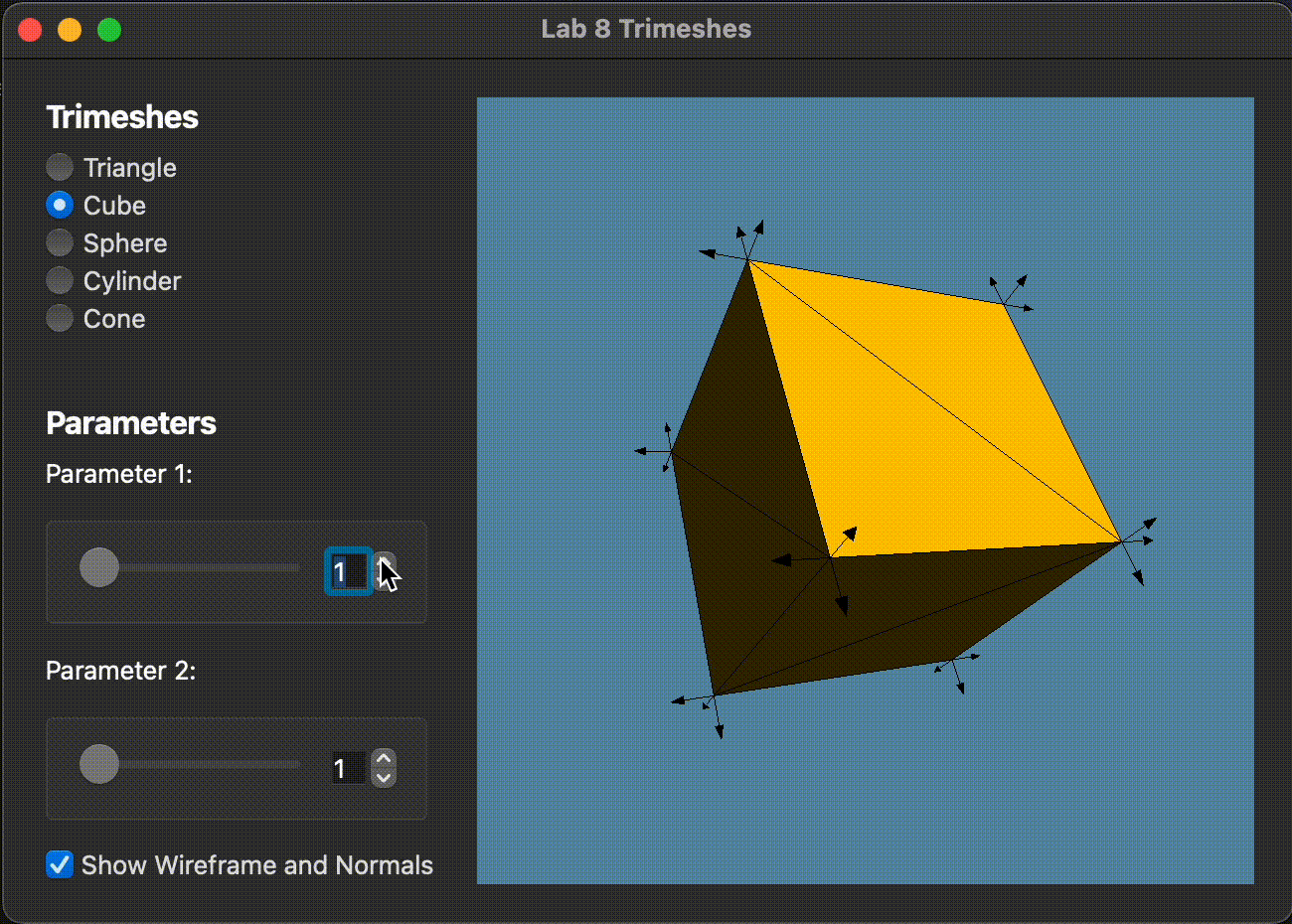Figure 14: Expected output of drawing a cube to the screen, at different levels of detail

## Spheres

Next, we'll set our sights on making a sphere.

This sphere's dimensions are identical to those of the implicit sphere from your raytracer. It is centered at the origin and has a radius of 0.5.

Caught on by now? All the trimesh shapes you'll make have the same dimensions as your implicit ones. This includes the cylinder and cone you'll implement in project 5.

But how do we tessellate a sphere? One way to do so is (bear with us) to think of a sphere like an orange. Oranges are can be sliced into wedges, and each wedge is made up segments. We can therefore build an orange (i.e. a sphere) by procedurally generating a collection of orange wedges.

Extra: this form of sphere tessellation seems familiar to me...

If you've any experience with 3D modelling software like Blender, you'll recognize this to be a UV-sphere, as distinct from an ico-sphere (which is based on subdividing an icosahedron). Here's a pretty good StackExchange discussion about them both.

We use a UV-sphere because it's good enough for our purposes, and it's fairly easy to program. If you're interested in learning about how an ico-sphere is made, you might be interested in searching up different methods for mesh subdivision. Incidentally, Loop subdivision is one of the mesh operations covered in CSCI 2240: Interactive Computer Graphics.Figure 15: Orange segments make up orange wedges, that in turn make up oranges.

This analogy is helpful for understanding the sphere's tessellation parameters. Parameter 1 controls the number of 'orange segments' (i.e. divisions in latitude), and parameter 2 controls the number of 'orange wedges' (i.e. divisions in longitude).

Refresher: what do latitude and longitude look like, again?

Incidentally, the grid lines in this image show what our sphere will look like, more or less: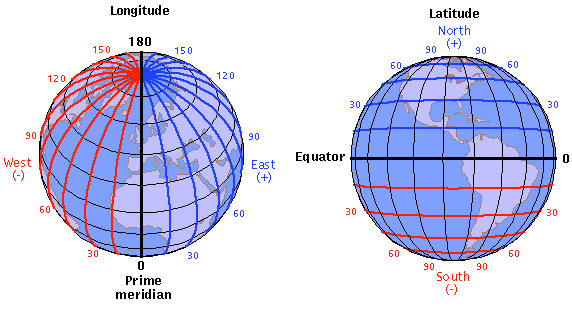Figure 16: Spheres faces are increasingly tessellated at higher levels of detail.

### Spherical Coordinates

We can represent this 'orange wedge and segment' idea using spherical coordinates. In terms of spherical coordinates, parameter 1 controls and parameter 2 controls .

Refresher: the spherical coordinate system

Remember polar coordinates from high school geometry? Spherical coordinates are like polar coordinates, but in 3D, with an added angle of elevation .

The spherical coordinate system thus specifies a 3D point in space using . for more details, please refer to this Wikipedia article on the Spherical Coordinate System.

### Wedge

Similar to making a cube, the first step in creating a sphere is to create a tile that can be replicated across the sphere, depending on the level of detail.

In Sphere.cpp, implement the makeTile() function. This function should update m_vertexData.

Remember: sphere normals are calculated differently than cube normals.

Once you've implemented makeTile(), you can use it to make a single wedge:

1. Uncomment the makeWedge() function call in setVertexData().
2. Implement the makeWedge() function using the makeTile() function you wrote.

Remember that we are making a wedge, so you need to pay attention to m_param1 and . Refer to the top half of Figure 16 for how m_param1 affects the level of detail. Also note that everything is in radians.

You might find the following functions helpful:

• float glm::radians(float x)
• float glm::sin(float x)
• float glm::cos(float x)

You might also find these equations useful:

• x = r * sin(phi) * sin(theta)
• y = r * cos(phi)
• z = r * sin(phi) * cos(theta)

Your single wedge should look like this: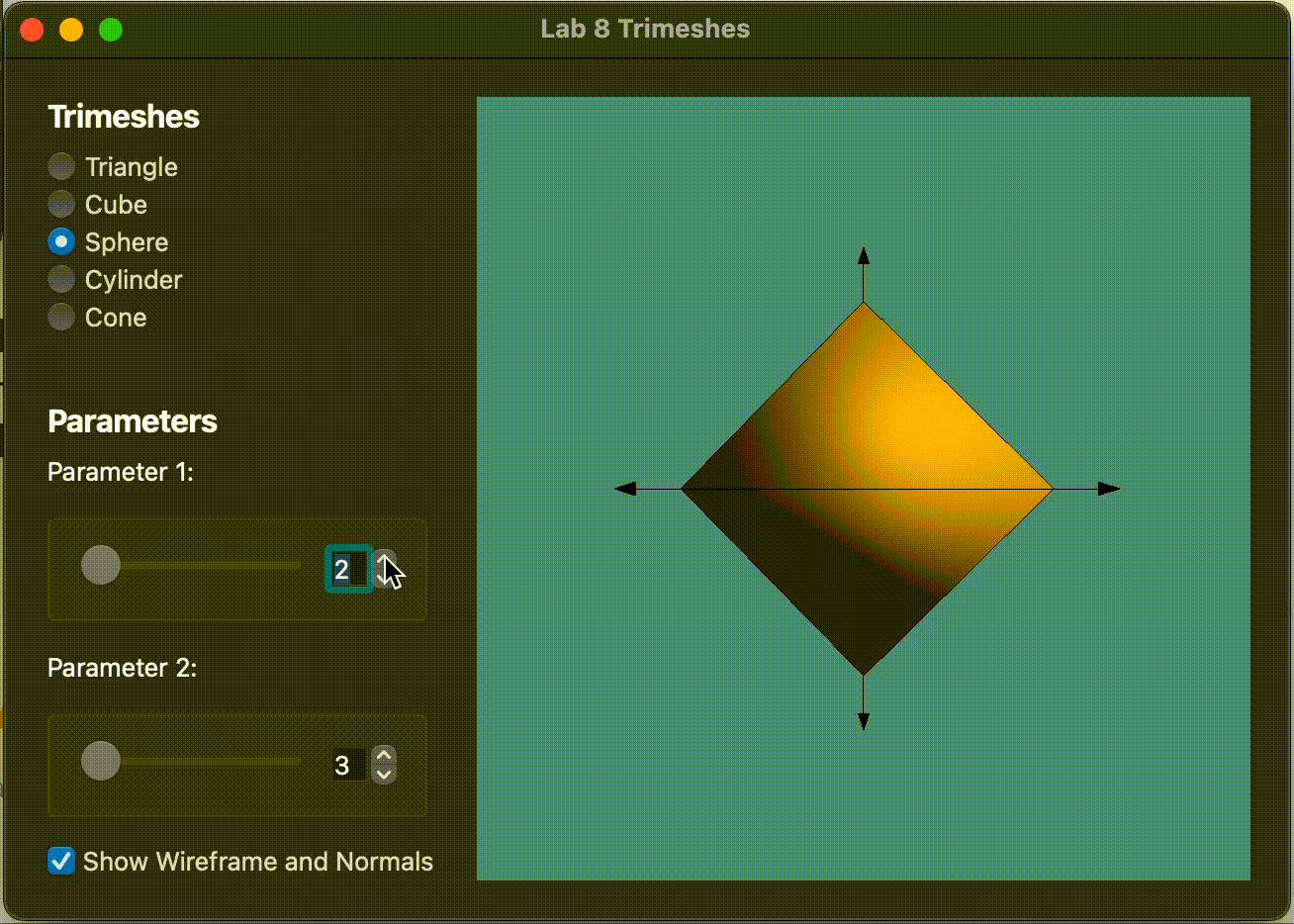Figure 19: Expected output of drawing a wedge to the screen, at different levels of detail.

### Full Spheres

We can now use our makeWedge() function to generate a full sphere!

1. Comment out the makeWedge() function call in setVertexData() and uncomment the makeSphere() function call.
2. Implement the makeSphere() function using makeWedge().

Remember that we are making multiple wedges (i.e. a sphere), so you need to pay attention to m_param2 and . Refer to the bottom half of Figure 16 for how m_param2 affects the level of detail.

Your sphere should look like this: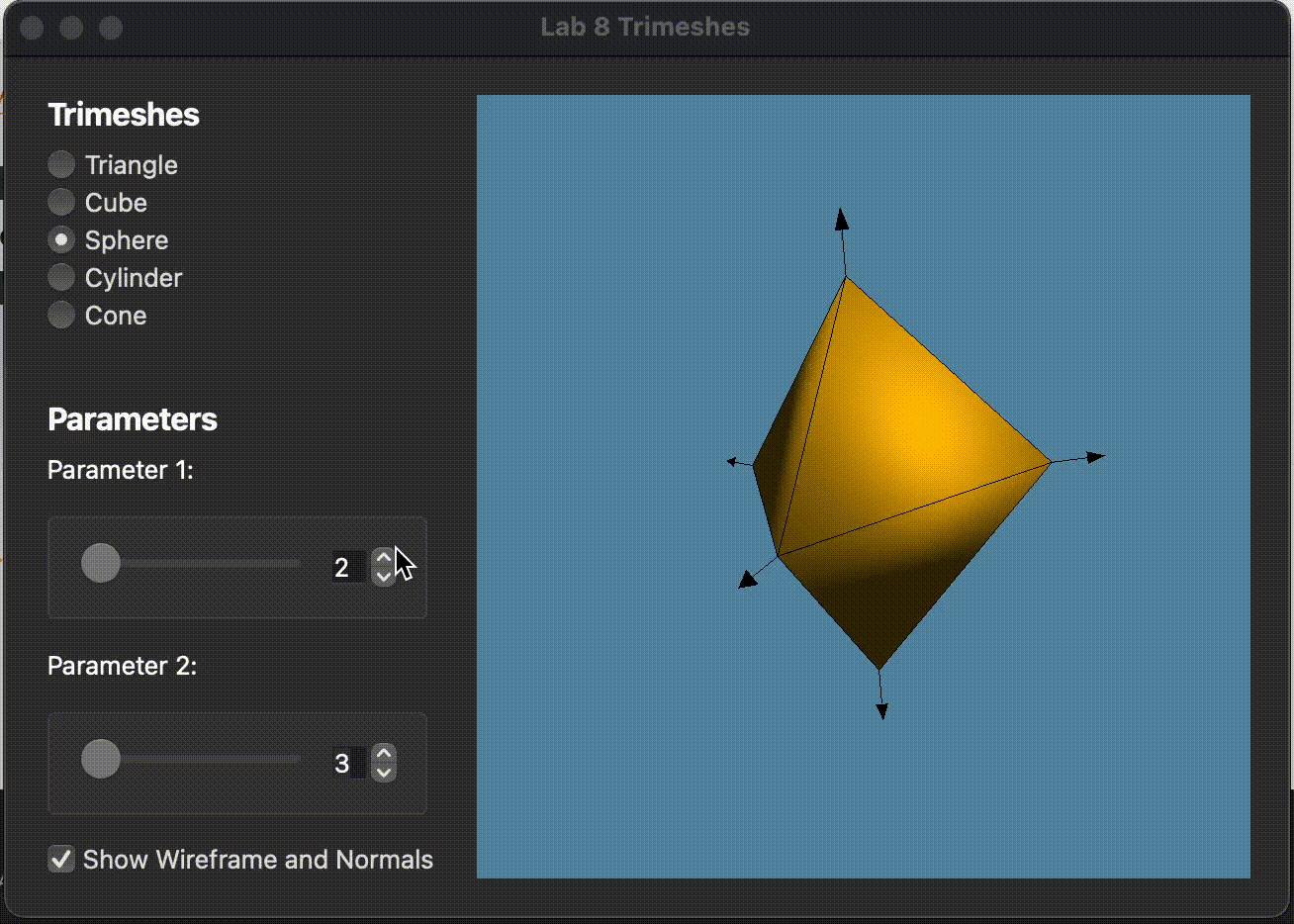Figure 20: Expected output of drawing a sphere to the screen, at different levels of detail.

## End

Congrats on finishing the Trimeshes lab! Now, it's time to submit your code and get checked off by a TA.

## Submission

Submit your Github repo for this lab to the "Lab 8: Trimeshes" assignment on Gradescope, then get checked off by a TA during lab hours.